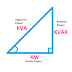KVAR is the unit of Reactive Power. The full form of KVAR is Kilo Volt Ampere Reactive. In an electrical AC power system, the electrical power can be measured in three formats - Active Power, Reactive Power, and Apparent Power. The concept of electrical power gives us the knowledge of the consumption of electricity by an electrical load. These three types of power come due to different types of load and their characteristics.

Active Power - Active power is also known as true power or real power because it is the actual power consumed by an electrical load. In an AC circuit, there is a concept of impedance and power factor. The active power depends upon the impedance of the load. The active power is measured in Watt or we can say watt is the unit of active power. When we talk about electrical power it only indicates the active power only.

Reactive Power - Reactive power is measured when there is any inductive or capacitive load. basically, reactive power is an imaginary power consumed by those inductive or capacitive loads. reactive power consumption happens when the voltage and current are not in the same phase. The inductive loads and capacitive loads cause to make phase differences between voltage and current. That is why reactive power consumption happens when there is inductive or capacitive load in the circuit. The reactive power is measured in VAR(volt-ampere-reactive). So, the KVAR is the bigger unit of reactive power.

Apparent Power - Apparent power is the simple measuring unit. It is independent of the types of load. Apparent power can be calculated by just multiplying the voltage and current. The apparent power is measured in VA(volt-ampere). Same as the bigger unit would be KVA or MVA.

KVAR Formula

As we know that KVAR is the unit of reactive power so we need to find out the reactive power formula. Before going to know the reactive power formula you should have a basic knowledge of phase difference and power factor.

The power factor is the cosine of the angle between voltage and current. For a resistive load, there is no phase difference between the voltage and current so the power factor will be unity. For an inductive load, the current lags behind the voltage so the power factor will be lagging. For a capacitive load, the current leads behind the voltage so the power factor will be leading.

The reactive power is denoted by Q and it can be calculated by this formula, Q = VI SinΦ

KVAR formula also as follows by Q = X*I*I

Here, X is the reactance of the circuit and the I is the current flow through the circuit.

For single-phase circuits,

Q(kVAR) = √(|S(kVA)|2 - P(kW)2)

For Three Phase Circuits,

(when Line to Line Voltage is available)

Q(kVAR) = √(|S(kVA)|2 - P(kW)2)

(when Line to Neutral Voltage is available)

Q(kVAR) = √(|S(kVA)|2 - P(kW)2)

KVAR calculation is very useful when we need to measure the reactive power of the circuit or compensate for the reactive power. The reactive power is balanced by using capacitor banks. So, in this case, we should measure reactive power for both the capacitor and circuit.

What is KVAR in Electrical? Full Form, FormulaReviewed by Author on April 22, 2022 Rating: 5